🦉 🎤   RapidMiner Wisdom 2020 - CALL FOR SPEAKERS   🦉 🎤

We are inviting all community members to submit proposals to speak at Wisdom 2020 in Boston.

Whether it's a cool RapidMiner trick or a use case implementation, we want to see what you have.
Form link is below and deadline for submissions is November 15. See you in Boston!

"Cross-Validation and its outputs in RM Studio"

12Administrator, Moderator, Employee, RapidMiner Certified Analyst, Community Manager, Member, University Professor, PM Moderator Posts: 2,525Community Manager
edited May 23

We get a lot of questions about how exactly the Cross Validation operator works, and what those 'exa', 'mod', and 'tes' outputs produce, in RM Studio. Hence it seems a good idea to explain this in depth as a KB article. So here I go...

What is Cross Validation?

Cross Validation is a method used to find a true estimate of the performance of mathematical models built on relatively small data sets. It builds a series of models on subsets of the data and tests each model on the remainder of the data to determine an average performance metric of the models, rather than one performance metric of one model.

Split Validation, a much simpler process where you just split the data once into a 'training' and 'testing' set, works very well for very large data sets. But it's Achilles heel is its assumption that the testing set is a representative sample of the entire data set. This is likely true for large data sets, but less and less likely the smaller your data set is.

How does Cross Validation work?

Cross Validation works by dividing the data set first into n partitions, and then building n unique models one at a time (each iteration is called a "fold"). For each iteration, a model is created using n-1 partitions and using the nth partition. The model itself is not relevant; what is retained is the model performance. This is repeated using a different partition as the hold-out set until each partition is used.

After the n performances are calculated, we calculate the average performance (and its standard deviation) of all n models built during the cross validation.

Lastly, we build a true model with the entire data set and display this calculated average performance.

Very Simplistic Example of Cross Validation

Let's walk through exactly how it works using a very simple example. Here is a data set where we have one independent variable (x) and one dependent variable (y). The goal is to produce the best linear regression model of these data using cross validation. The y values were randomly generated.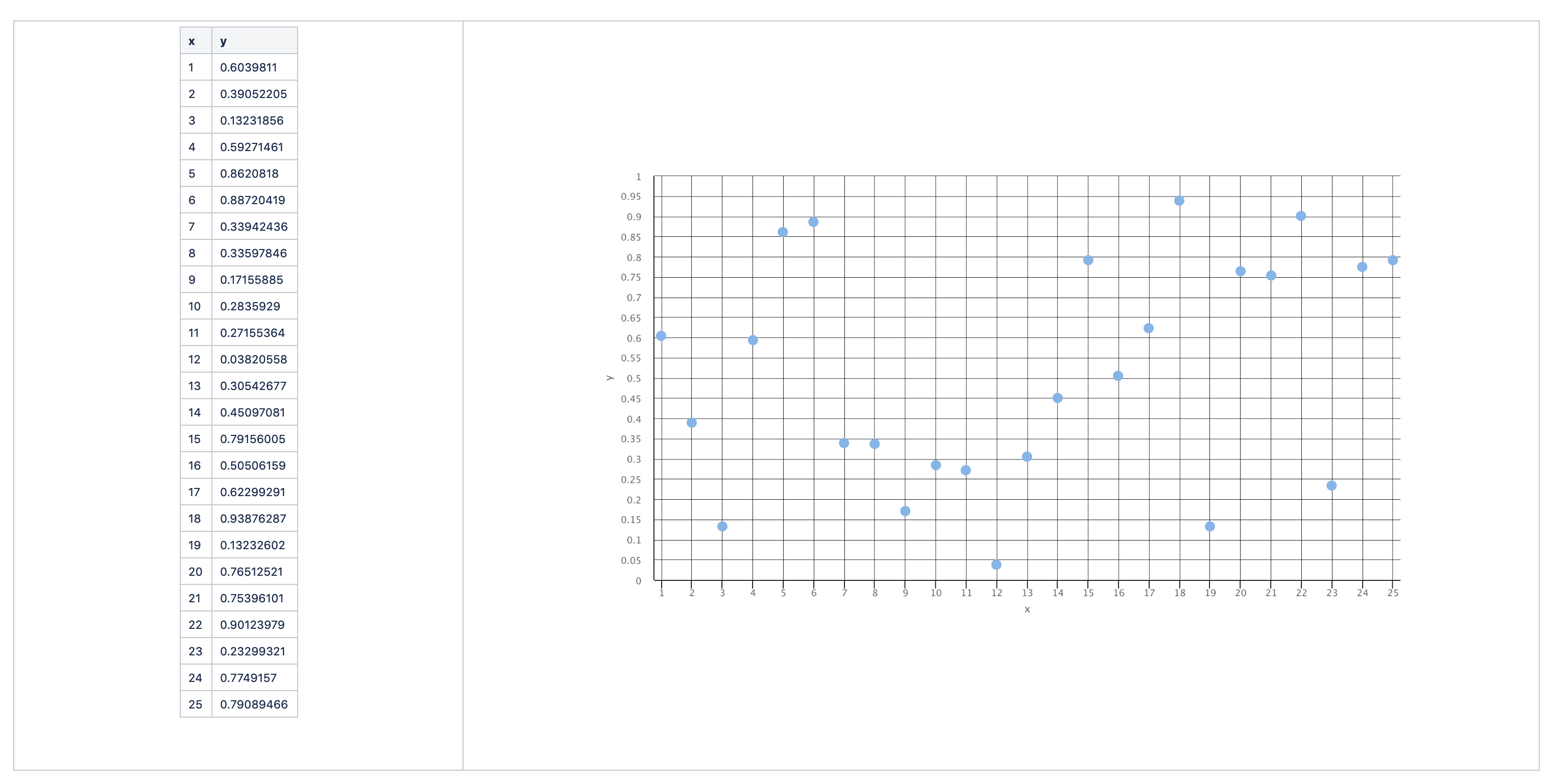In this example we're going to use linear sampling5-fold cross validation, and RSME as our performance metric:

• Linear sampling - we will pull our hold-out sets in order which the data sets is given to us
• 5-fold Cross Validation - we will perform five iterations to find five performances using five hold-out sets
• RSME - the root-mean-squared-error of the predicted values of each hold-out set vs the actual values

Fold 1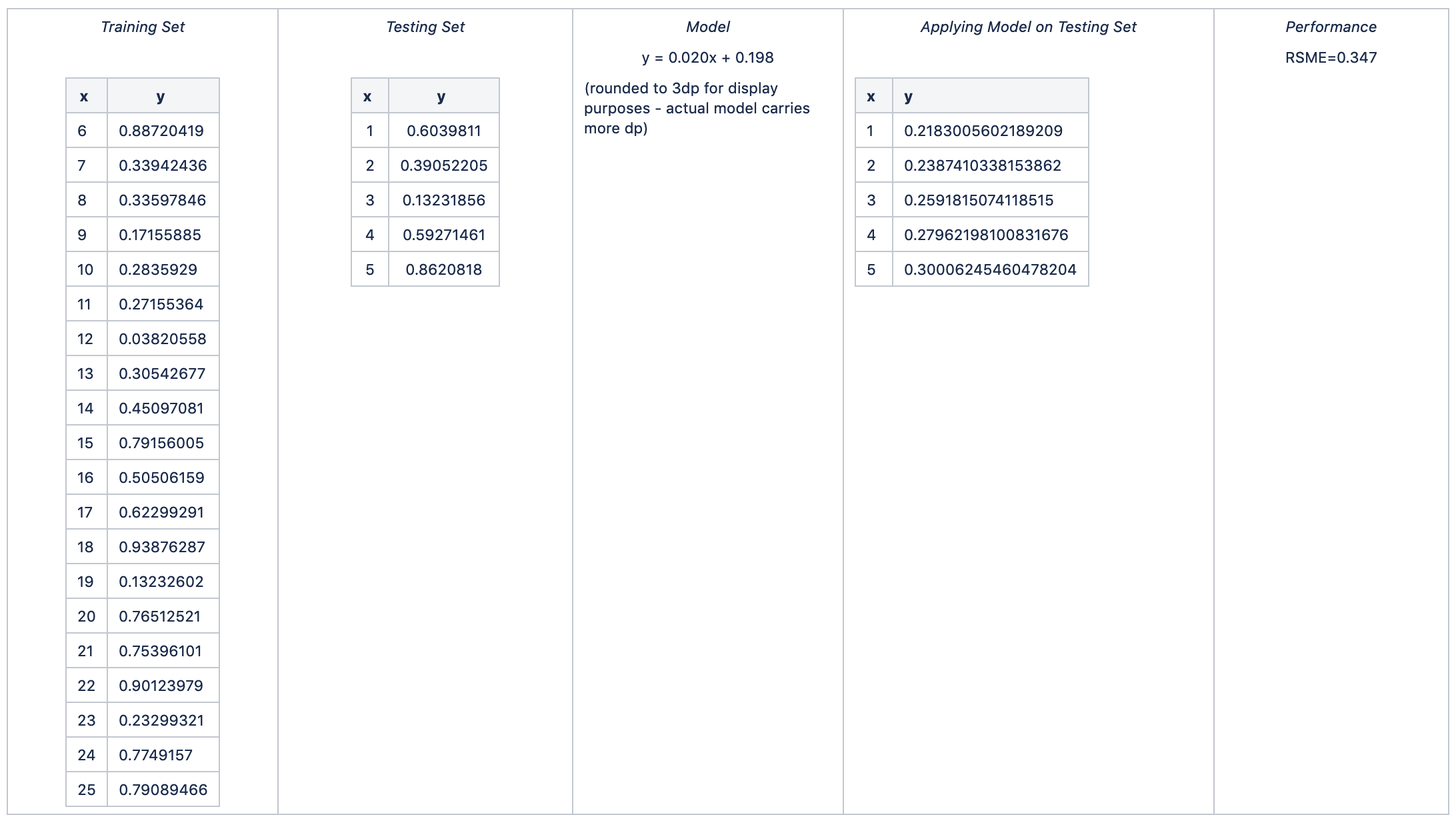Fold 2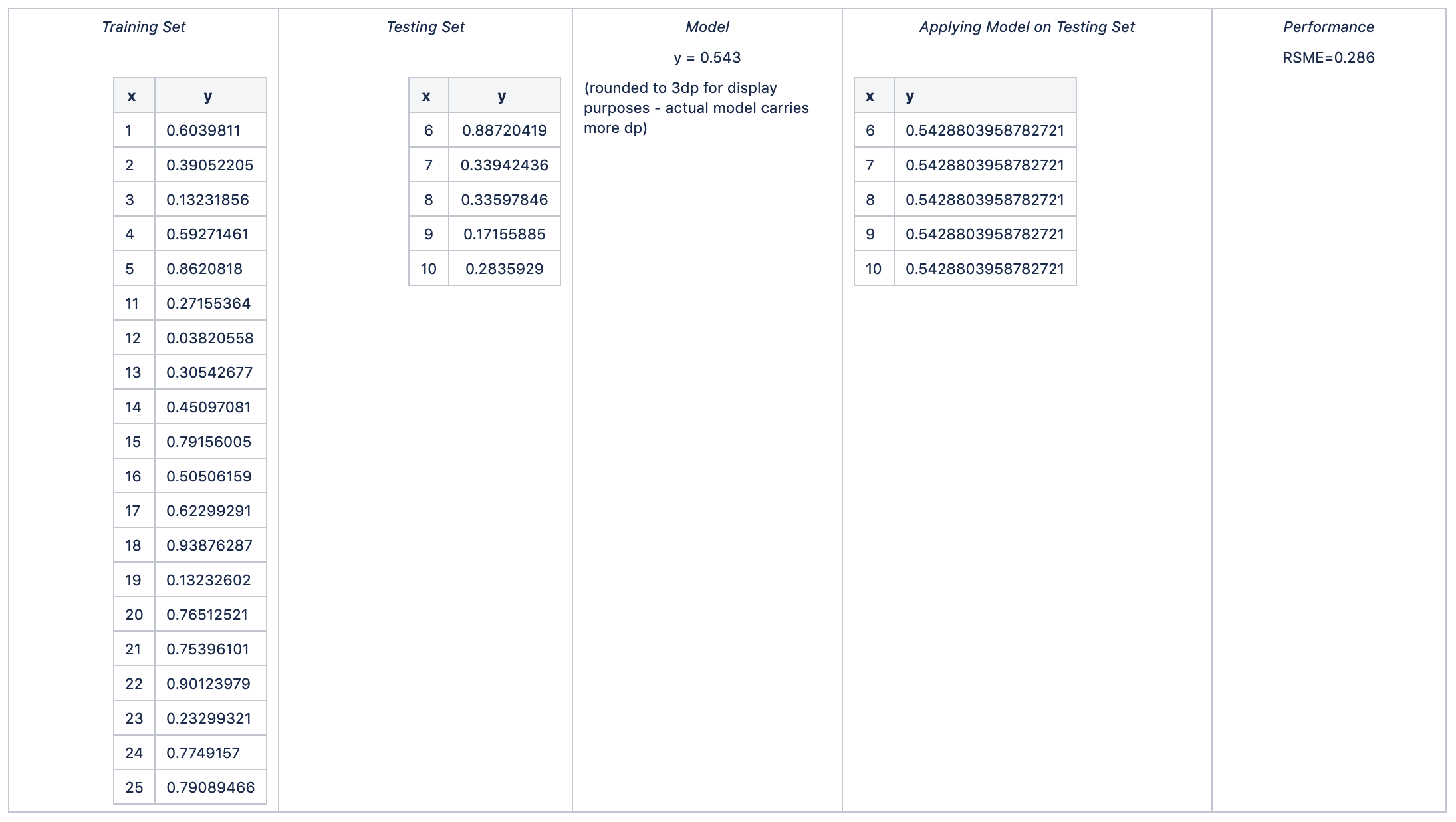Fold 3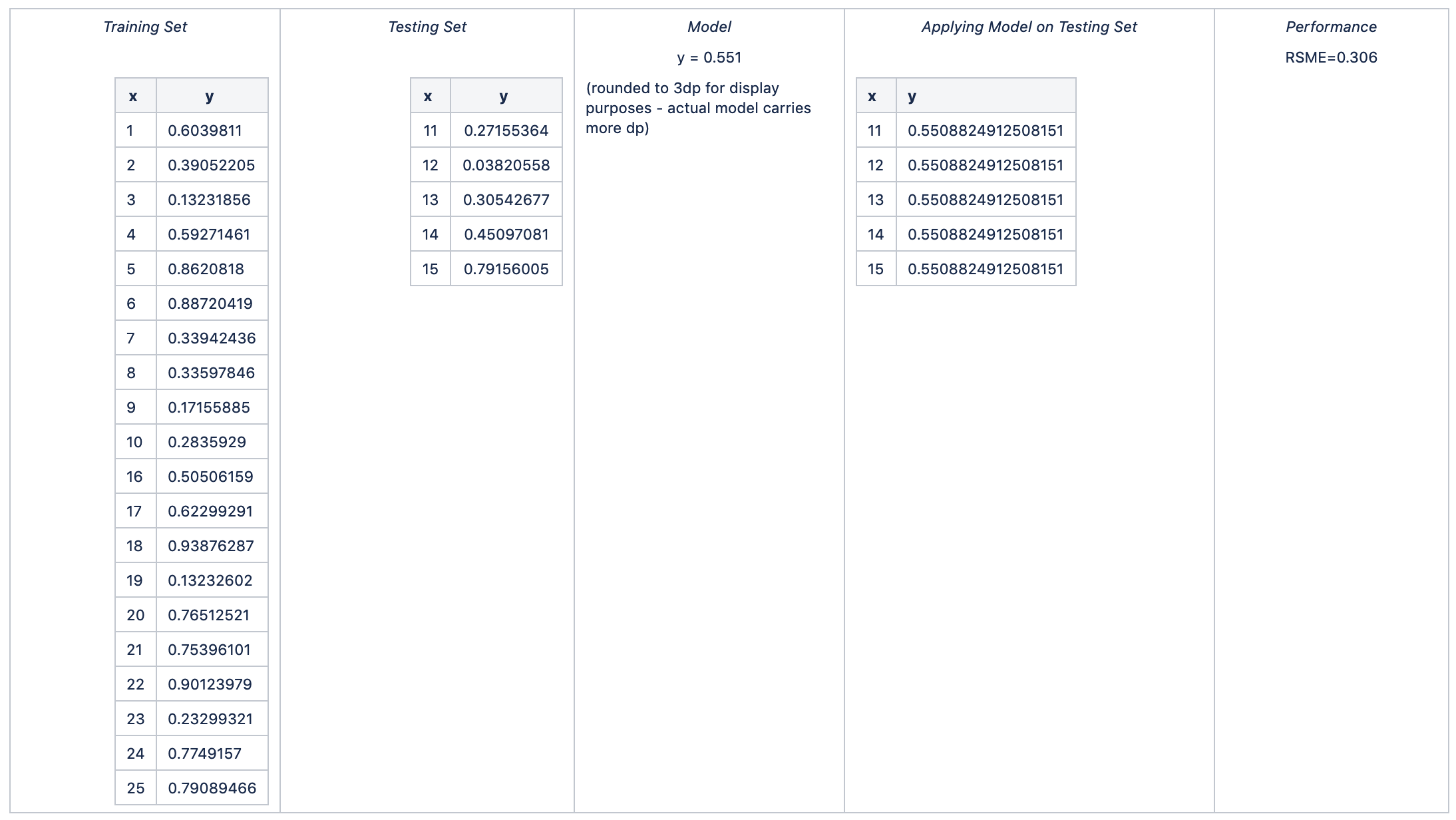Fold 4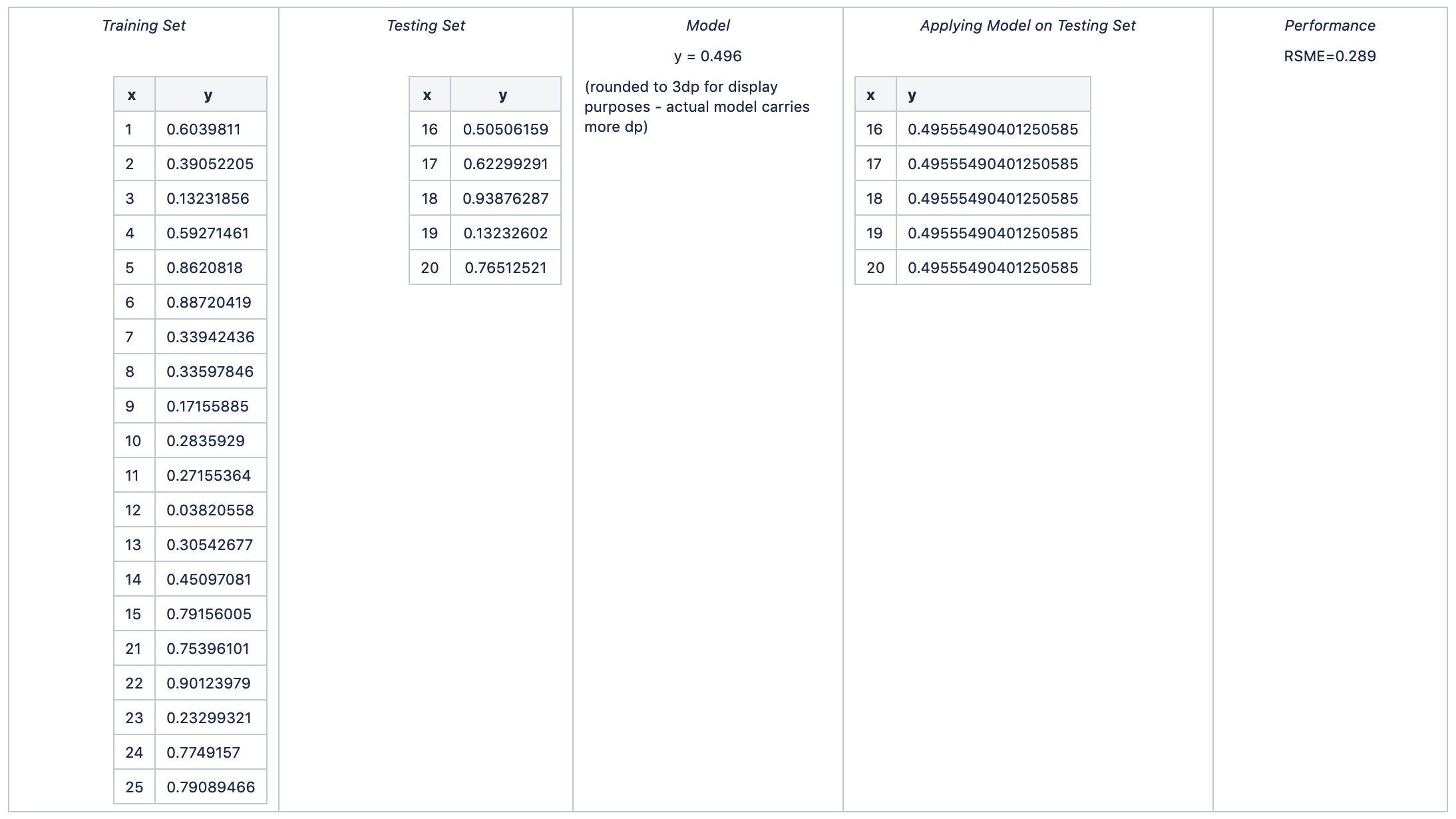Fold 5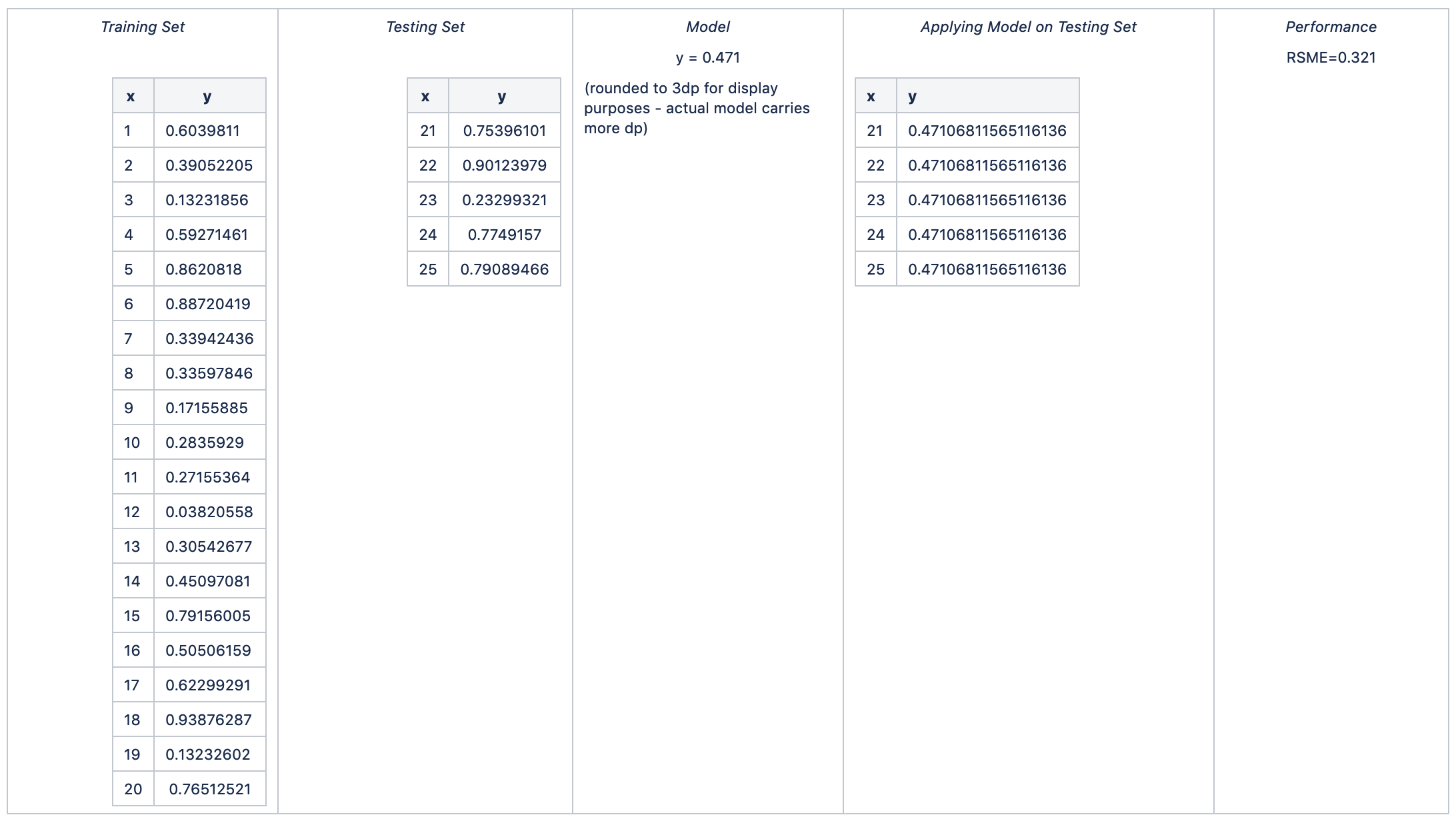Hence:

average RSME =  avg (0.347, 0.286, 0.306, 0.289, 0.321) = 0.310

standard deviation of RSME = stdev (0.347, 0.286, 0.306, 0.289, 0.321) = 0.025

The model representing this performance metric is none of the models shown above; it is a new model produced on the entire data set. Sometimes this is considered the "n+1" th iteration.

Calculate the actual model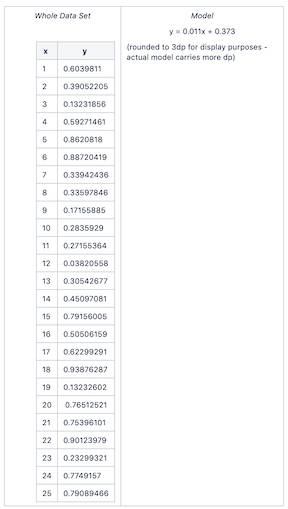What are the outputs of the Cross Validation operator in RapidMiner Studio?

Ok now we can answer this question! They are as follows:

• mod - the actual mathematical model built on the entire data set (see last step above). Usually only the relevant coefficients or equivalent are displayed.
• exa - the original ExampleSet (data). This is simple a pass-thru.
• tes - the testing ExampleSet. Note that this is NOT the application of the final model on the data set; rather it displays all the hold-out testing sets from the n-fold cross validation in one appended list.
• per = the average performance of the n-fold cross validation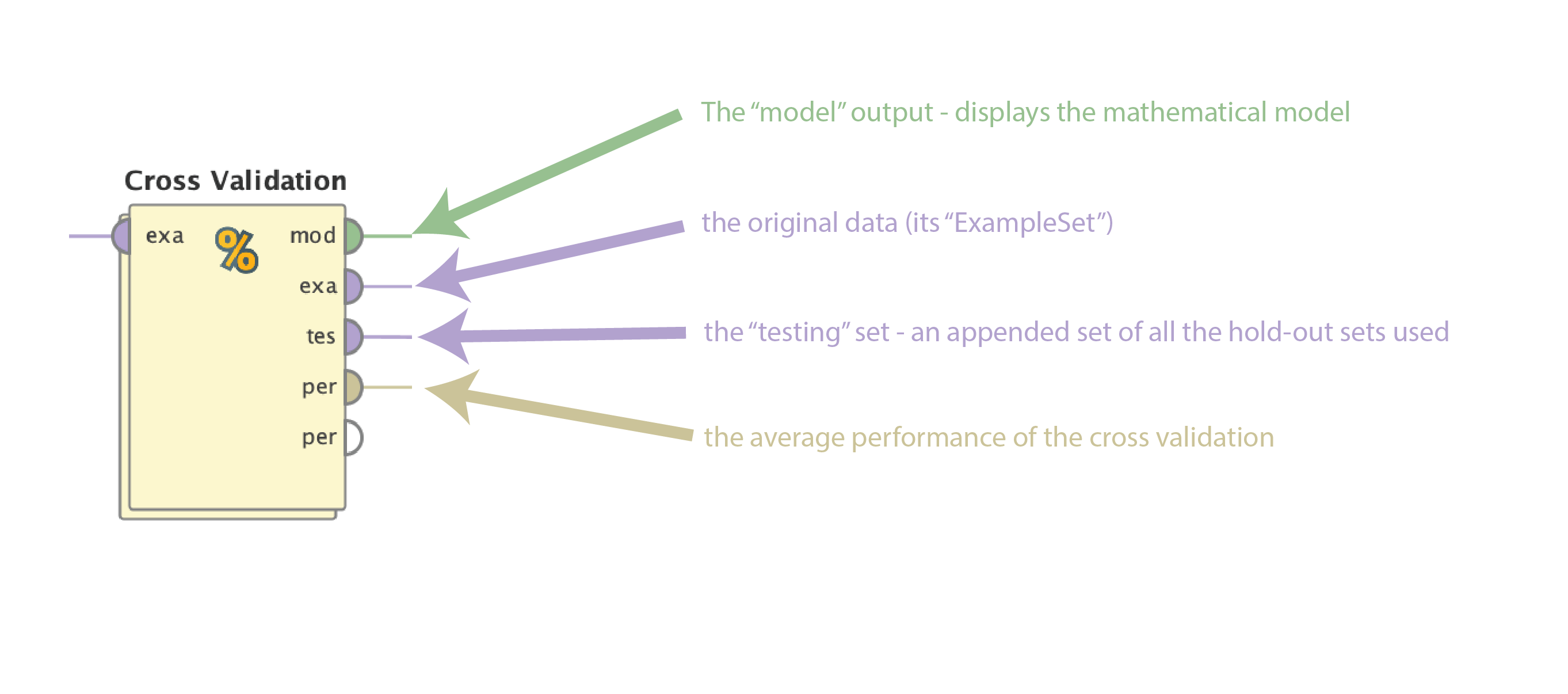For our example, these are as follows: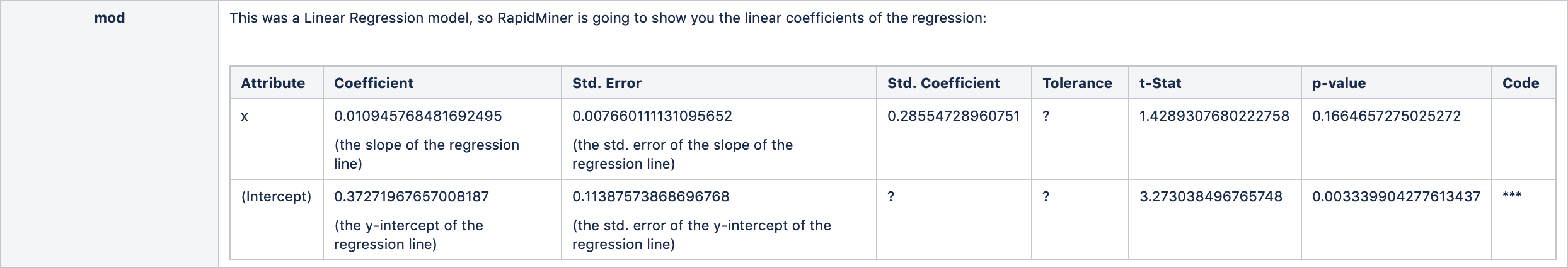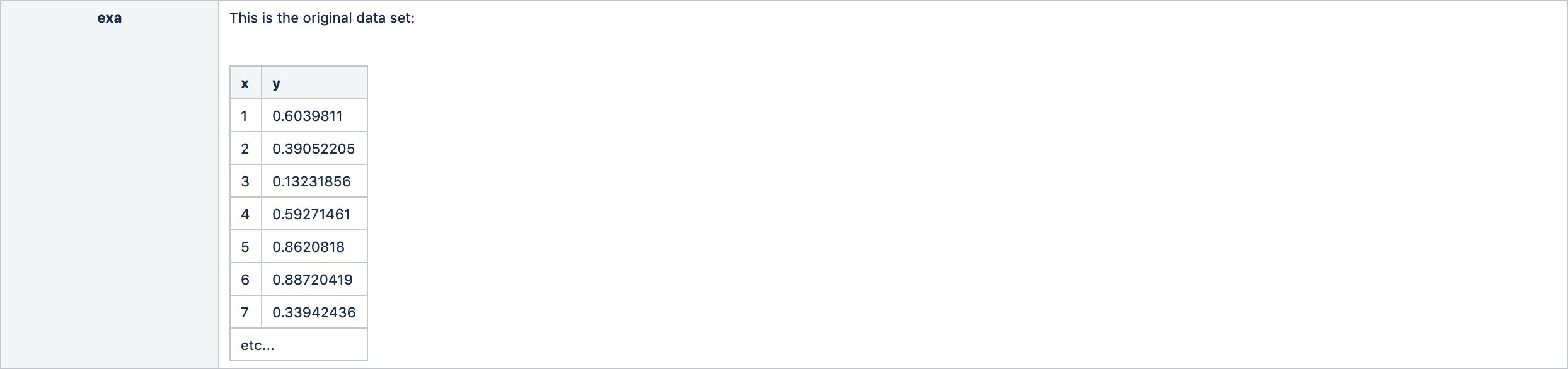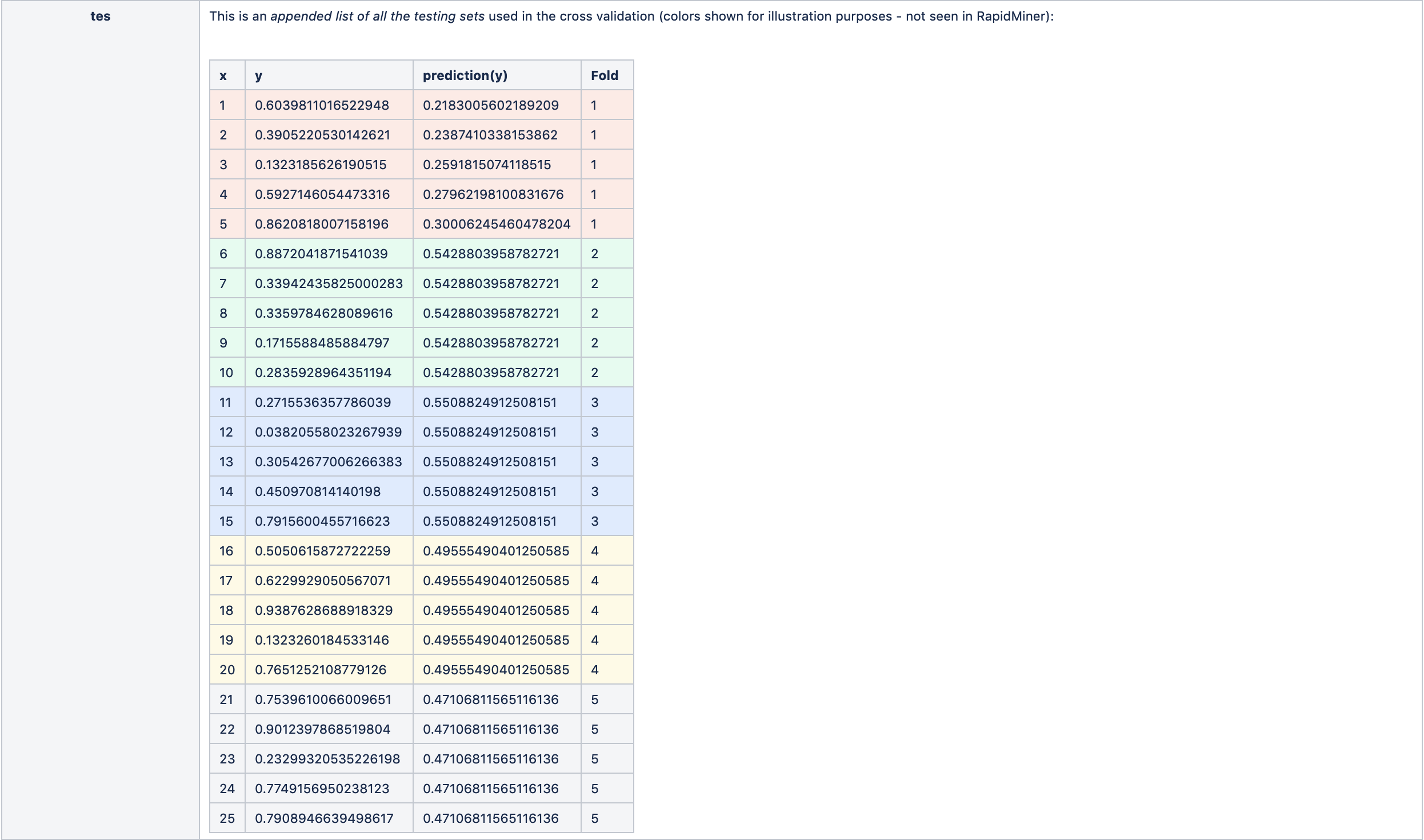Why should we go through all this trouble?

I am going to just insert a few paragraphs from founder @IngoRM here:

Cross validation is not about model building at all.  It is a common scheme to estimate (not calculate! - subtle but important difference) how well a given model will work on unseen data.  So the fact that we deliver a model at the end (for convenience reasons) might lead you to the conclusion that it actually is about model building as well - but this is just not the case.

Ok, here is why this validation is an approximation of an estimation for a given model only: typically you want to use as much data as possible since labeled data is expensive and in most cases the learning curves show you that more data leads to better models.  So you build your model on the complete data set since you hope this is the best model you can get.  Brilliant!  This is the given model from above.  You could now gamble and use this model in practice, hoping for the best.  Or you want to know in advance if this model is really good before you use it in practice.  I prefer the latter approach ;-)

So only now (actually kind of after you built the model on all data) you are of course also interested in learning how well this model works in practice on unseen data.  Well, the closest estimate you could do is a so-called leave-one-our validation where you use all but 1 data points for training and the one you left out for testing.  You repeat this for all data points.  This way, the models you built are "closest" to the one you are actually interested in (since only one example is missing) but unfortunately this approach is not feasible for most real-world scenarios since you would need to build 1,000,000 models for a data set with 1,000,000 examples.

Here is where cross-validation enters the stage.  It is just a more feasible approximation of something which already was only an estimation to begin with (since we omitted one example even in the LOO case).  But this is still better than nothing.  The important thing is: It is a performance estimation for the original model (built on all data), and not a tool for model selection.  If at all, you could misuse a cross-validation as a tool for example selection but I won't go into this discussion now.

More food for thought: you might have an idea how to average 10 linear regression models - what do we do with 10 neural networks with different optimized network structures?  Or 10 different decisions trees?  How to average those?  In general this problem can not be solved anyway.

The net is that none of these are good ideas; you should do the right thing which is to build one model on as much data as you can and use cross-validation to estimate how well this model will perform on new data.

Hope this helps some people. Thank you @yyhuang and @IngoRM for your help!

Scott
----------------------
Don't forget to submit your great ideas for Wisdom 2020! Deadline is November 15.

Wisdom 2020 – Call for Speakers FormTagged: